Life In 19x19http://lifein19x19.com/ KataGo @ Igo Hatsuyoron 120http://lifein19x19.com/viewtopic.php?f=18&t=16995 Page 5 of 6

 Author: Cassandra [ Mon Jan 11, 2021 10:34 am ] Post subject: Re: KataGo @ Igo Hatsuyoron 120 "Classical" way of play => Black's weakness in the upper left (contd.)Black has to passively back away (II). Click Here To Show Diagram Code`[go]\$\$Wcm108\$\$ +---------------------------------------+\$\$ | . . . . . X . O . . . . X . . . O X . |\$\$ | . . . . X O O . O O . X O X O . . O . |\$\$ | . . . X O X X O . . . X O . O O O . . |\$\$ | O X O , . X O . . O . X . O O X . . . |\$\$ | . 4 X X X X O O O . O X X X X X O O . |\$\$ | . X . O O O X X X O . . X . X O . . . |\$\$ | O O O O . X O O X X O O . O X . O O . |\$\$ | O X . X . X . O O X O . . . X X X X X |\$\$ | O X . X . . . O X X O . X X X X . O O |\$\$ | X O O X . X . O X O O X X O O O O . . |\$\$ | X X O O X . . O X O . X O O O . X X X |\$\$ | X X . O X . . O X O . X O O X X X O O |\$\$ | X X O O X . . O X O . X O . O . X X O |\$\$ | X O O X . X . O X O . X X O O O X O O |\$\$ | X O X . X X . O X O X . O X X X O O O |\$\$ | O X X X X . . O X O O O O X O X O O X |\$\$ | O . . . O X . O X X X X X . O X X O X |\$\$ | O . X . . . X O O O O O O X O X . O X |\$\$ | . X . . . . . O . . . . O X X X . O . |\$\$ +---------------------------------------+[/go]`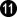connects immediately, seeling the lock at the left side. Click Here To Show Diagram Code`[go]\$\$Wcm112\$\$ +---------------------------------------+\$\$ | . . . . a X . O . . . . X . . . O X . |\$\$ | . . . 1 X O O . O O . X O X O . . O . |\$\$ | . . . X O X X O . . . X O . O O O . . |\$\$ | O X O , 2 X O . . O . X . O O X . . . |\$\$ | . X X X X X O O O . O X X X X X O O . |\$\$ | . X . O O O X X X O . . X . X O . . . |\$\$ | O O O O . X O O X X O O . O X . O O . |\$\$ | O X . X . X . O O X O . . . X X X X X |\$\$ | O X . X . . . O X X O . X X X X . O O |\$\$ | X O O X . X . O X O O X X O O O O . . |\$\$ | X X O O X . . O X O . X O O O . X X X |\$\$ | X X . O X . . O X O . X O O X X X O O |\$\$ | X X O O X . . O X O . X O . O . X X O |\$\$ | X O O X . X . O X O . X X O O O X O O |\$\$ | X O X . X X . O X O X . O X X X O O O |\$\$ | O X X X X . . O X O O O O X O X O O X |\$\$ | O . . . O X . O X X X X X . O X X O X |\$\$ | O . X . . . X O O O O O O X O X . O X |\$\$ | . X . . . . . O . . . . O X X X . O . |\$\$ +---------------------------------------+[/go]`But White remembers the dud at the right, and gives atari with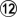.It is best for Black to capture with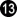.Connecting at a, instead, might turn the entire corner into a seki. Click Here To Show Diagram Code`[go]\$\$Wcm112\$\$ +---------------------------------------+\$\$ | . 6 5 . 7 X 9 O . . . . X . . . O X . |\$\$ | . 4 3 O X O O . O O . X O X O . . O . |\$\$ | . . . X 8 X X O . . . X O . O O O . . |\$\$ | O X O , X X O . . O . X . O O X . . . |\$\$ | . X X X X X O O O . O X X X X X O O . |\$\$ | . X . O O O X X X O . . X . X O . . . |\$\$ | O O O O . X O O X X O O . O X . O O . |\$\$ | O X . X . X . O O X O . . . X X X X X |\$\$ | O X . X . . . O X X O . X X X X . O O |\$\$ | X O O X . X . O X O O X X O O O O . . |\$\$ | X X O O X . . O X O . X O O O . X X X |\$\$ | X X . O X . . O X O . X O O X X X O O |\$\$ | X X O O X . . O X O . X O . O . X X O |\$\$ | X O O X . X . O X O . X X O O O X O O |\$\$ | X O X . X X . O X O X . O X X X O O O |\$\$ | O X X X X . . O X O O O O X O X O O X |\$\$ | O . . . O X . O X X X X X . O X X O X |\$\$ | O . X . . . X O O O O O O X O X . O X |\$\$ | . X . . . . . O . . . . O X X X . O . |\$\$ +---------------------------------------+[/go]`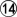extends into the corner.Blocking at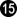is Black's only choice to prevent a seki in the corner.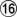prepares creating a ko shape at the upper edge.It is best for Black to connect with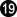, as he will be unable to win a ko fight in this corner. Click Here To Show Diagram Code`[go]\$\$Wcm120\$\$ +---------------------------------------+\$\$ | . X O 4 O 3 O O . . . . X . . . O X . |\$\$ | . X O O X O O . O O . X O X O . . O . |\$\$ | . . 2 X X X X O . . . X O . O O O . . |\$\$ | O X O , X X O . . O . X . O O X . . . |\$\$ | . X X X X X O O O . O X X X X X O O . |\$\$ | . X . O O O X X X O . . X . X O . . . |\$\$ | O O O O . X O O X X O O . O X . O O . |\$\$ | O X . X . X . O O X O . . . X X X X X |\$\$ | O X . X . . . O X X O . X X X X . O O |\$\$ | X O O X . X . O X O O X X O O O O . . |\$\$ | X X O O X . . O X O . X O O O . X X X |\$\$ | X X . O X . . O X O . X O O X X X O O |\$\$ | X X O O X . . O X O . X O . O . X X O |\$\$ | X O O X . X . O X O . X X O O O X O O |\$\$ | X O X . X X . O X O X . O X X X O O O |\$\$ | O X X X X . . O X O O O O X O X O O X |\$\$ | O . . . O X . O X X X X X . O X X O X |\$\$ | O . X . . a X O O O O O O X O X . O X |\$\$ | . X . . . . . O . . . . O X X X . O . |\$\$ +---------------------------------------+[/go]`After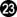, the upper left corner is settled.White will continue with a in the lower left corner.Compared to the superordinate variation above, Black gained one point in the upper left corner, but White did not lose a tempo in the Main Semeai there.The net effect is zero, so White will win this variation by FOUR points, too.

 Author: Cassandra [ Mon Jan 11, 2021 11:57 am ] Post subject: Re: KataGo @ Igo Hatsuyoron 120 "Classical" way of play => Black's weakness in the upper left (contd.)Black gives atari at the left edge. Click Here To Show Diagram Code`[go]\$\$Wcm108\$\$ +---------------------------------------+\$\$ | . . . . . X . O . . . . X . . . O X . |\$\$ | . . . . X O O . O O . X O X O . . O . |\$\$ | a . . X O X X O . . . X O . O O O . . |\$\$ | O X O , . X O . . O . X . O O X . . . |\$\$ | 4 . X X X X O O O . O X X X X X O O . |\$\$ | . X . O O O X X X O . . X . X O . . . |\$\$ | O O O O . X O O X X O O . O X . O O . |\$\$ | O X . X . X . O O X O . . . X X X X X |\$\$ | O X . X . . . O X X O . X X X X . O O |\$\$ | X O O X . X . O X O O X X O O O O . . |\$\$ | X X O O X . . O X O . X O O O . X X X |\$\$ | X X . O X . . O X O . X O O X X X O O |\$\$ | X X O O X . . O X O . X O . O . X X O |\$\$ | X O O X . X . O X O . X X O O O X O O |\$\$ | X O X . X X . O X O X . O X X X O O O |\$\$ | O X X X X . . O X O O O O X O X O O X |\$\$ | O . . . O X . O X X X X X . O X X O X |\$\$ | O . X . . . X O O O O O O X O X . O X |\$\$ | . X . . . . . O . . . . O X X X . O . |\$\$ +---------------------------------------+[/go]`gives atari from the outside.You will easily realise that starting with the inside atari at a results in a change in the order of moves with the sequence shown below. Click Here To Show Diagram Code`[go]\$\$Wcm108\$\$ +---------------------------------------+\$\$ | . . . . . X . O . . . . X . . . O X . |\$\$ | . . . . X O O . O O . X O X O . . O . |\$\$ | 6 5 . X O X X O . . . X O . O O O . . |\$\$ | O X O , . X O . . O . X . O O X . . . |\$\$ | X . X X X X O O O . O X X X X X O O . |\$\$ | . X . O O O X X X O . . X . X O . . . |\$\$ | O O O O . X O O X X O O . O X . O O . |\$\$ | O X . X . X . O O X O . . . X X X X X |\$\$ | O X . X . . . O X X O . X X X X . O O |\$\$ | X O O X . X . O X O O X X O O O O . . |\$\$ | X X O O X . . O X O . X O O O . X X X |\$\$ | X X . O X . . O X O . X O O X X X O O |\$\$ | X X O O X . . O X O . X O . O . X X O |\$\$ | X O O X . X . O X O . X X O O O X O O |\$\$ | X O X . X X . O X O X . O X X X O O O |\$\$ | O X X X X . . O X O O O O X O X O O X |\$\$ | O . . . O X . O X X X X X . O X X O X |\$\$ | O . X . . . X O O O O O O X O X . O X |\$\$ | . X . . . . . O . . . . O X X X . O . |\$\$ +---------------------------------------+[/go]`gives counter-atari in the corner.Black has to capture with. Click Here To Show Diagram Code`[go]\$\$Wcm108\$\$ +---------------------------------------+\$\$ | . . . . . X . O . . . . X . . . O X . |\$\$ | 7 . 8 a X O O . O O . X O X O . . O . |\$\$ | X O . X O X X O . . . X O . O O O . . |\$\$ | 9 X O , . X O . . O . X . O O X . . . |\$\$ | X 0 X X X X O O O . O X X X X X O O . |\$\$ | . X . O O O X X X O . . X . X O . . . |\$\$ | O O O O . X O O X X O O . O X . O O . |\$\$ | O X . X . X . O O X O . . . X X X X X |\$\$ | O X . X . . . O X X O . X X X X . O O |\$\$ | X O O X . X . O X O O X X O O O O . . |\$\$ | X X O O X . . O X O . X O O O . X X X |\$\$ | X X . O X . . O X O . X O O X X X O O |\$\$ | X X O O X . . O X O . X O . O . X X O |\$\$ | X O O X . X . O X O . X X O O O X O O |\$\$ | X O X . X X . O X O X . O X X X O O O |\$\$ | O X X X X . . O X O O O O X O X O O X |\$\$ | O . . . O X . O X X X X X . O X X O X |\$\$ | O . X . . . X O O O O O O X O X . O X |\$\$ | . X . . . . . O . . . . O X X X . O . |\$\$ +---------------------------------------+[/go]`gives another atari in the corner.Black has to defend his cutting point at a with.captures the first ko.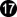connects the second one, as Black cannot afford to fight any ko in this corner, due to White's unbeatable ko threats in the hanezeki. Click Here To Show Diagram Code`[go]\$\$Wcm118\$\$ +---------------------------------------+\$\$ | . . . . . X . O . . . . X . c . O X . |\$\$ | O . X . X O O . O O 1 X O X O . . O . |\$\$ | . O . X O X X O . . . X O b O O O . . |\$\$ | O X O , a X O . . O . X . O O X . . . |\$\$ | X X X X X X O O O . O X X X X X O O . |\$\$ | . X . O O O X X X O . . X . X O . . . |\$\$ | O O O O . X O O X X O O . O X . O O . |\$\$ | O X . X . X . O O X O . . . X X X X X |\$\$ | O X . X . . . O X X O . X X X X . O O |\$\$ | X O O X . X . O X O O X X O O O O . . |\$\$ | X X O O X . . O X O . X O O O . X X X |\$\$ | X X . O X . . O X O . X O O X X X O O |\$\$ | X X O O X . . O X O . X O . O . X X O |\$\$ | X O O X . X . O X O . X X O O O X O O |\$\$ | X O X . X X . O X O X . O X X X O O O |\$\$ | O X X X X . . O X O O O O X O X O O X |\$\$ | O . . . O X . O X X X X X . O X X O X |\$\$ | O . X . . . X O O O O O O X O X . O X |\$\$ | . X . . . . . O . . . . O X X X . O . |\$\$ +---------------------------------------+[/go]`White continues with the Main Semeai.She has created a shortage of liberties for Black's upper left corner, and so will keep Black down at par with the Punishment Semeai Liberty Level.Please note that Black is unable to remove his shortage of liberties by capturing at a, because this move would lose him a very decisive tempo in the Main Semeai.Quite similar, White must not mistakenly play at b or c at the right (which usually would be answered by Black to avoid losing points), because Black would tenuki here to a in the upper left corner, making the game countable. Click Here To Show Diagram Code`[go]\$\$Bcm151\$\$ +---------------------------------------+\$\$ | . . . . . X . O . . . . X . . . O X . |\$\$ | O . X . X O O . O O O X O X O . . O . |\$\$ | 5 O . X O X X O . . . X O . O O O . . |\$\$ | O X O 4 . X O . . O . X 2 O O X . . . |\$\$ | X X X X X X O O O . O X X X X X O O . |\$\$ | 1 X 3 O O O X X X O . . X . X O . . . |\$\$ | O O O O . X O O X X O O . O X . O O . |\$\$ | O X . X . X . O O X O . . . X X X X X |\$\$ | O X . X . . . O X X O . X X X X . O O |\$\$ | X O O X . X . O X O O X X O O O O . . |\$\$ | X X O O X . . O X O . X O O O . X X X |\$\$ | X X . O X . . O X O . X O O X X X O O |\$\$ | X X O O X . . O X O . X O . O . X X O |\$\$ | X O O X . X . O X O . X X O O O X O O |\$\$ | X O X . X X . O X O X . O X X X O O O |\$\$ | O X X X X . . O X O O O O X O X O O X |\$\$ | O . . . O X . O X X X X X 6 O X X O X |\$\$ | O . X . . . X O O O O O O X O X . O X |\$\$ | . X . . . . . O . . . . O X X X . O . |\$\$ +---------------------------------------+[/go]`Black will occupy White's liberties in the upper left last.Please note that I did not adjust the diagram, just assume that this sequence is played earlier.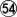is atari, also threatening a snap-back, so Black has to capture the ko at the left edge with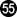.White uses the capture of the hanezeki's tail as a ko threat. Click Here To Show Diagram Code`[go]\$\$Bcm157\$\$ +---------------------------------------+\$\$ | . . . . . X . O . . . . X . . . O X . |\$\$ | O . X . X O O . O O O X O X O . . O . |\$\$ | X O . X O X X O . . . X O . O O O . . |\$\$ | 2 X O O a X O . . O . X O O O X . . . |\$\$ | X X X X X X O O O . O X X X X X O O . |\$\$ | X X X O O O . . . O . . X . X O . . . |\$\$ | O O O O . X O O . . O O . O X . O O . |\$\$ | O X . X . X . O O . O . . . X X X X X |\$\$ | O X . X . . . O . . O . X X X X . O O |\$\$ | X O O X . X . O . O O X X O O O O . . |\$\$ | X X O O X . . O . O . X O O O . X X X |\$\$ | X X . O X . . O . O . X O O X X X O O |\$\$ | X X O O X . . O . O . X O . O . X X O |\$\$ | X O O X . X . O . O . X X O O O X O O |\$\$ | X O X . X X . O . O X . O X X X O O O |\$\$ | O X X X X . . O . O O O O X O X O O X |\$\$ | O . . . O X . O . . . . 1 O O X X O X |\$\$ | O . X . . . X O O O O O O X O X . O X |\$\$ | . X . . . . . O . . . . O X X X . O . |\$\$ +---------------------------------------+[/go]`Black has to recapture with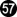, if he wants that his right side survives.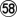recaptures the ko in the upper left, and there is nothing Black can do to stop White from killing his upper left corner by capturing at a with her next move.White will win the game by a large margin.

 Author: Cassandra [ Mon Jan 11, 2021 2:37 pm ] Post subject: Re: KataGo @ Igo Hatsuyoron 120 "Classical" way of play => Black's weakness in the upper left (contd.)Black descends to the left edge. Click Here To Show Diagram Code`[go]\$\$Wcm108\$\$ +---------------------------------------+\$\$ | . . . . . X . O . . . . X . . . O X . |\$\$ | . . . . X O O . O O . X O X O . . O . |\$\$ | . . . X O X X O . . . X O . O O O . . |\$\$ | O X O , . X O . . O . X . O O X . . . |\$\$ | . . X X X X O O O . O X X X X X O O . |\$\$ | 4 X . O O O X X X O . . X . X O . . . |\$\$ | O O O O . X O O X X O O . O X . O O . |\$\$ | O X . X . X . O O X O . . . X X X X X |\$\$ | O X . X . . . O X X O . X X X X . O O |\$\$ | X O O X . X . O X O O X X O O O O . . |\$\$ | X X O O X . . O X O . X O O O . X X X |\$\$ | X X . O X . . O X O . X O O X X X O O |\$\$ | X X O O X . . O X O . X O . O . X X O |\$\$ | X O O X . X . O X O . X X O O O X O O |\$\$ | X O X . X X . O X O X . O X X X O O O |\$\$ | O X X X X . . O X O O O O X O X O O X |\$\$ | O . . . O X . O X X X X X . O X X O X |\$\$ | O . X . . . X O O O O O O X O X . O X |\$\$ | . X . . . . . O . . . . O X X X . O . |\$\$ +---------------------------------------+[/go]`descends to the left edge, also intending to maximise the corner territory. Click Here To Show Diagram Code`[go]\$\$Wcm112\$\$ +---------------------------------------+\$\$ | . . . . 5 X . O . . . . X . . . O X . |\$\$ | . . 4 3 X O O . O O . X O X O . . O . |\$\$ | . 1 . X O X X O . . . X O . O O O . . |\$\$ | O X O , a X O . . O . X . O O X . . . |\$\$ | . 2 X X X X O O O . O X X X X X O O . |\$\$ | X X . O O O X X X O . . X . X O . . . |\$\$ | O O O O . X O O X X O O . O X . O O . |\$\$ | O X . X . X . O O X O . . . X X X X X |\$\$ | O X . X . . . O X X O . X X X X . O O |\$\$ | X O O X . X . O X O O X X O O O O . . |\$\$ | X X O O X . . O X O . X O O O . X X X |\$\$ | X X . O X . . O X O . X O O X X X O O |\$\$ | X X O O X . . O X O . X O . O . X X O |\$\$ | X O O X . X . O X O . X X O O O X O O |\$\$ | X O X . X X . O X O X . O X X X O O O |\$\$ | O X X X X . . O X O O O O X O X O O X |\$\$ | O . . . O X . O X X X X X . O X X O X |\$\$ | O . X . . . X O O O O O O X O X . O X |\$\$ | . X . . . . . O . . . . O X X X . O . |\$\$ +---------------------------------------+[/go]`First, White gives atari with, forcing Black to connect at.Thereafter, Whitecuts at the top, also giving atari.Black has to give counter-atari with.Capturing with a, or connecting at 116, instead, would result in a real desaster in the corner.captures Black's stone in the corner, so gaining a massive profit from reducing Black's top left corner. Click Here To Show Diagram Code`[go]\$\$Wcm112\$\$ +---------------------------------------+\$\$ | . . . . O X . O . . . . X . . . O X . |\$\$ | . 6 X O . O O . O O . X O X O . . O . |\$\$ | . O . X O X X O . . . X O . O O O . . |\$\$ | O X O , . X O . . O . X . O O X . . . |\$\$ | . X X X X X O O O . O X X X X X O O . |\$\$ | X X . O O O X X X O . . X . X O . . . |\$\$ | O O O O . X O O X X O O . O X . O O . |\$\$ | O X . X . X . O O X O . . . X X X X X |\$\$ | O X . X . . . O X X O . X X X X . O O |\$\$ | X O O X . X . O X O O X X O O O O . . |\$\$ | X X O O X . . O X O . X O O O . X X X |\$\$ | X X . O X . . O X O . X O O X X X O O |\$\$ | X X O O X . . O X O . X O . O . X X O |\$\$ | X O O X . X . O X O . X X O O O X O O |\$\$ | X O X . X X . O X O X . O X X X O O O |\$\$ | O X X X X . . O X O O O O X O X O O X |\$\$ | O . . . O X . O X X X X X . O X X O X |\$\$ | O . X . . a X O O O O O O X O X . O X |\$\$ | . X . . . . . O . . . . O X X X . O . |\$\$ +---------------------------------------+[/go]`is necessary to bring Black's corner to life.White will continue with the usual endgame in the lower left, starting with a.White will win the game by about 12 points.

 Author: Cassandra [ Tue Jan 12, 2021 12:57 am ] Post subject: Re: KataGo @ Igo Hatsuyoron 120 "Classical" way of play => Black's weakness in the upper left (contd.)KataGo does not want to follow Yamada's original sequence any longer!When painting the diagrams for my postings for LifeIn19x19, I suddenly had the strange feeling that something was wrong.Finally, I found out: In my draft for the update of our website, I had used another order of moves than had been originally shown by Yamada Shinji to us.And now, with KataGo's new net, it does not want at all to follow that line of play... Click Here To Show Diagram Code`[go]\$\$Wcm98\$\$ +---------------------------------------+\$\$ | . . . . c b . . . . . . X . . . O X . |\$\$ | . . . . 4 3 5 X O O . X O X O . . O . |\$\$ | . . . . . X a O . . . X O . O O O . . |\$\$ | . X O , . . . . . O . X . O O X . . . |\$\$ | . . X X X X O O O . O X X X X X O O . |\$\$ | . X . O O O X X X O . . X . X O . . . |\$\$ | O O O O . X O O X X O O . O X . 1 O . |\$\$ | O X . X . X . O O X O . . . X X X X X |\$\$ | O X . X . . . O X X O . X X X X . O O |\$\$ | X O O X . X . O X O O X X O O O O . . |\$\$ | X X O O X . . O X O . X O O O . X X X |\$\$ | X X . O X . . O X O . X O O X X X O O |\$\$ | X X O O X . . O X O . X O . O . X X O |\$\$ | X O O 2 O X . O X O . X X O O O X O O |\$\$ | X O X O X X . O X O X . O X X X O O O |\$\$ | O X X X X . . O X O O O O X O X O O X |\$\$ | O . . . O X . O X X X X X . O X X O X |\$\$ | O . X . . . X O O O O O O X O X . O X |\$\$ | . X . . . . . O . . . . O X X X . O . |\$\$ +---------------------------------------+[/go]`(Again,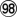for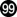is inserted for simplicity.)After White's draw-back to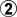, KataGo's new net does not seem to consider Yamada's move at a any longer for Black.Instead, KataGo favours the immediate hane at b, and the descent to c, with the latter getting the upper hand over time. Click Here To Show Diagram Code`[go]\$\$Wcm98\$\$ +---------------------------------------+\$\$ | . . . . . 8 . a . . . . X . . . O X . |\$\$ | . . . . X O O X O O . X O X O . . O . |\$\$ | . . . 9 7 X 6 O . . . X O . O O O . . |\$\$ | . X O , . . . . . O . X . O O X . . . |\$\$ | . . X X X X O O O . O X X X X X O O . |\$\$ | . X . O O O X X X O . . X . X O . . . |\$\$ | O O O O . X O O X X O O . O X . O O . |\$\$ | O X . X . X . O O X O . . . X X X X X |\$\$ | O X . X . . . O X X O . X X X X . O O |\$\$ | X O O X . X . O X O O X X O O O O . . |\$\$ | X X O O X . . O X O . X O O O . X X X |\$\$ | X X . O X . . O X O . X O O X X X O O |\$\$ | X X O O X . . O X O . X O . O . X X O |\$\$ | X O O X . X . O X O . X X O O O X O O |\$\$ | X O X . X X . O X O X . O X X X O O O |\$\$ | O X X X X . . O X O O O O X O X O O X |\$\$ | O . . . O X . O X X X X X . O X X O X |\$\$ | O . X . . . X O O O O O O X O X . O X |\$\$ | . X . . . . . O . . . . O X X X . O . |\$\$ +---------------------------------------+[/go]`If we let Black play the "original"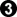, White will still cut at, but after Black's atari with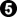, she does NOT capture at a!KataGo's new net draws back to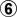, instead. sacrificing White's two stones at the upper edge. Click Here To Show Diagram Code`[go]\$\$Wcm106\$\$ +---------------------------------------+\$\$ | . . . . . X 2 . . . . . X . . . O X . |\$\$ | . . 4 6 X O O X O O . X O X O . . O . |\$\$ | . 3 5 O O X X O . . . X O . O O O . . |\$\$ | . X O , . . . . . O . X . O O X . . . |\$\$ | . . X X X X O O O . O X X X X X O O . |\$\$ | . X . O O O X X X O . . X . X O . . . |\$\$ | O O O O . X O O X X O O . O X . O O . |\$\$ | O X . X . X . O O X O . . . X X X X X |\$\$ | O X . X . . . O X X O . X X X X . O O |\$\$ | X O O X . X . O X O O X X O O O O . . |\$\$ | X X O O X . . O X O . X O O O . X X X |\$\$ | X X . O X . . O X O . X O O X X X O O |\$\$ | X X O O X . . O X O . X O . O . X X O |\$\$ | X O O X . X . O X O . X X O O O X O O |\$\$ | X O X . X X . O X O X . O X X X O O O |\$\$ | O X X X X . . O X O O O O X O X O O X |\$\$ | O . . . O X . O X X X X X . O X X O X |\$\$ | O . X . . . X O O O O O O X O X . O X |\$\$ | . X . . . . . O . . . . O X X X . O . |\$\$ +---------------------------------------+[/go]`Blacktakes White's two stones off the board. I suppose that this has to be done anyway -- sooner or later.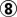blocks in the corner, whereafter Black attacks White's shape from above. Click Here To Show Diagram Code`[go]\$\$Wcm112\$\$ +---------------------------------------+\$\$ | . . . . . X X . . . . . X . . . O X . |\$\$ | 7 6 X X X . . X O O . X O X O . . O . |\$\$ | . O O O O X X O . . . X O . O O O . . |\$\$ | 1 X O , 5 3 4 . . O . X . O O X . . . |\$\$ | . 2 X X X X O O O . O X X X X X O O . |\$\$ | . X . O O O X X X O . . X . X O . . . |\$\$ | O O O O . X O O X X O O . O X . O O . |\$\$ | O X . X . X . O O X O . . . X X X X X |\$\$ | O X . X . . . O X X O . X X X X . O O |\$\$ | X O O X . X . O X O O X X O O O O . . |\$\$ | X X O O X . . O X O . X O O O . X X X |\$\$ | X X . O X . . O X O . X O O X X X O O |\$\$ | X X O O X . . O X O . X O . O . X X O |\$\$ | X O O X . X . O X O . X X O O O X O O |\$\$ | X O X . X X . O X O X . O X X X O O O |\$\$ | O X X X X . . O X O O O O X O X O O X |\$\$ | O . . . O X . O X X X X X . O X X O X |\$\$ | O . X . . . X O O O O O O X O X . O X |\$\$ | . X . . . . . O . . . . O X X X . O . |\$\$ +---------------------------------------+[/go]`White's atari athas to be answered by the connection of.Now, the time is ripe to cut Black apart withat the right.Black continues in the corner withJumping to 118, instead, would not lead to a better result for him. Click Here To Show Diagram Code`[go]\$\$Wcm118\$\$ +---------------------------------------+\$\$ | . . . . . X X . . . . . X . . . O X . |\$\$ | O X X X X . . X O O . X O X O . . O . |\$\$ | 5 O O O O X X O . 8 . X O . O O O . . |\$\$ | O X O a O O X 2 6 O . X . O O X . . . |\$\$ | 4 X X X X X O O O 7 O X X X X X O O . |\$\$ | 3 X 9 O O O X X X O . . X . X O . . . |\$\$ | O O O O . X O O X X O O . O X . O O . |\$\$ | O X . X . X . O O X O . . . X X X X X |\$\$ | O X . X . . . O X X O . X X X X . O O |\$\$ | X O O X . X . O X O O X X O O O O . . |\$\$ | X X O O X . . O X O . X O O O . X X X |\$\$ | X X . O X . . O X O . X O O X X X O O |\$\$ | X X O O X . . O X O . X O . O . X X O |\$\$ | X O O X . X . O X O . X X O O O X O O |\$\$ | X O X . X X . O X O X . O X X X O O O |\$\$ | O X X X X . . O X O O O O X O X O O X |\$\$ | O . . . O X . O X X X X X . O X X O X |\$\$ | O . X . . . X O O O O O O X O X . O X |\$\$ | . X . . . . . O . . . . O X X X . O . |\$\$ +---------------------------------------+[/go]`It is best for Black now to push withat the top.As a matter of course, he could capture White's stones in the upper left corner with B a, W 122, B 121.However, he would be forced to really take White's group at the left off the board, enabling White to win the Punishment Semeai at the right.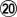captures Black's partial group in the upper left, while Black starts capturing White's top with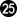.This exchange favours White, who will win the game by about 15 points.Please note that the hanezeki will remain untouched here, as both large groups live independently.

 Author: hakuseki [ Tue Jan 12, 2021 6:10 am ] Post subject: Re: KataGo @ Igo Hatsuyoron 120 Is there any hint of when this network will be available for download? I'd very much like to play with it.

 Author: Cassandra [ Tue Jan 12, 2021 8:09 am ] Post subject: Re: KataGo @ Igo Hatsuyoron 120 hakuseki wrote:Is there any hint of when this network will be available for download? I'd very much like to play with it.According to Friday9i's current schedule, he intends to train his new network for additional three months, at least, before a potential publication.I have the feeling that he is currently extremely concerned about (probably somewhat unusual) "white spots" in the variation tree of the problem, which KataGo has not yet sufficiently explored / analysed during training. If I understood that issue correct, he is still on the way do develop some kind of tool, which should help a lot to overcome that problem.To give you a recent example:As far as I remember (disclaimer: my memory can be deceptive; probably there had been no need to go there any deeper), Lightvector's net did not have any problems with the original line of play after Yamada's tsuke in the upper left.Probably, Friday9i's new net dislikes this order of moves now, because it had (additionally) analysed subvariations after Joachim's (yet unpubished; this is part of the draft I mentioned above) trial to "punish" KataGo's immediate crosscut (no Black guzumi played before) during training. In my understanding, the resulting positions have significant similarities, especially with regard to the hidden weaknesses in Black's corner.

 Author: Cassandra [ Tue Jan 12, 2021 8:18 am ] Post subject: Re: KataGo @ Igo Hatsuyoron 120 "Classical" way of play => Black's weakness in the upper left (contd.)KataGo does not want to follow Yamada's original sequence any longer!I feel that I have to add this posting to make clear that Yamada Shinji 6p must not be blamed for the abovementioned impact of KataGo's analysis.When he, together with his study group, worked out his improvement over the "classical" atari, he had been asked only, if he could be so very kind to validate the correctness of the guzumi.At that time, the endgame at the left side of the board was still played "late", i.e. after the dissolving of the hanezeki, i.e. after the occupation of White's liberties in the upper left.Once that border of Black's upper left corner is settled, KataGo's new finding is NOT applicable any longer!It was Prof. Jeong, who told us later that it would be technically correct to start that endgame early.Therefore, it were some poor amateurs, who overlooked the hidden weaknesses of Black's upper left corner, when transposing Yamada's sequence to an earlier moment in the game.

 Author: Friday9i [ Tue Jan 12, 2021 9:00 am ] Post subject: Re: KataGo @ Igo Hatsuyoron 120 hakuseki wrote:Is there any hint of when this network will be available for download? I'd very much like to play with it.HiAs said by Cassandra, I effectively intend to release the new net once the training is finished, in a few months. It already is very significantly stronger than g146 : -): it beats it from starting position even if 50x less visits, as it improved its understanding and knows how to squeeze a bit more profit. From a panel of thousands of random Igo Hatsuyoron positions, with only 25 visits, it manages to beat g146 with 100 visits, with an about 40 Elo performance (from about same Elo 2 months ago), so it still improves quite healthily. I'm also developing a small python tool to automate exploration of board positions (using KataGo's analysis engine), which will hopefully help wide exploration of the problem, and possibly help it to discover unexpected moves : -). We'll see in the coming weeks if we get some interesting results from this additional exploration.Thanks to Cassandra for its panel of Igo positions (very precious for training), and big thank you to lightvector for precious advice to launch the run with adequate training parameters : -)

 Author: Cassandra [ Fri Nov 19, 2021 8:38 am ] Post subject: Re: KataGo @ Igo Hatsuyoron 120 Harry drew my attention to the following Chinese video about KataGo's solution to Igo Hatsuyôron 120:https://www.bilibili.com/video/BV1JC4y1x7Uv/The comments are in Chinese AND ENGLISH.

 Author: Cassandra [ Fri Nov 19, 2021 11:24 pm ] Post subject: Re: KataGo @ Igo Hatsuyoron 120 2021 update of Igo Hatsuyôron 120 website uploaded!The variation trees for the new sections can be accessed via ...http://igohatsuyoron120.de/2015/0058.htm andhttp://igohatsuyoron120.de/2015/0513b.htmYou will find a short description of the update's topics at the top of these pages.----------Please let me know if you find any issues.

Author:  Friday9i [ Thu Feb 10, 2022 3:53 pm ]
Post subject:  Re: KataGo @ Igo Hatsuyoron 120

Dear all,

I'm happy to share the result of 11-months of training a new KataGo version to (probably) solve Igo Hatsuyoron 120 problem!!!

This is probably the most difficult Go problem ever created, see wikipedia for the historical context: https://en.wikipedia.org/wiki/Igo_Hatsuy%C5%8Dron

This was done with the incredible help from Thomas (Cassandra here, one of the very best world experts of this problem) and David (lightvector), creator of KataGo:
- Thomas helped a lot by providing many new positions to submit to KataGo to train it. This was an interactive and crucial activity: I regularly sent him new and better versions of KataGo, he identified weaknesses and misunderstanding, provided new positions around these issues, KataGo selfplayed these positions and eventually understood them! Until Thomas found new weaknesses.
- David not only created KataGo, but he also helped me a lot to setup the run, provide the initial panel of positions, adjust the parameters, debug some difficult technical issues and gave me precious advice all along
I used 2 RTX GPUs (3080+2070) during most of these 11-months of selfplay + training KataGo.

The best 40b network is derived from 40b-s5G09 (best net of g170's run, June 2020): this net was already very strong (only ~250 Elo weaker than current best kata1 40b network) and able to play many rule sets: Japanese, Chinese, Tromp-Taylor, New-Zealand...
You will find several details on this run in the attached pdf: process, intermediate results, final results, impact of komi and rule sets, etc.

The latest 40b network is linked in the file, and the direct link is: https://magentacloud.de/s/8i9BmTSKjf2ipCr
Igo Hatsuyoron 120's initial position is available as an sgf included in the pdf (in Annex A).

I hope you'll enjoy this marvelous problem, best regards!
Friday9i

PS: I just updated the file to correct a small detail (the first 50 downloads contain an incorrect date, no big deal)

 Attachments:Igo Hatsuyoron 120 - KataGo g170 run - v2.pdf [843.89 KiB] Downloaded 718 times

 Author: gaius [ Fri Feb 11, 2022 1:31 pm ] Post subject: Re: KataGo @ Igo Hatsuyoron 120 This looks completely amazing, I just spent some time going over some of the SGF's in this thread and the problem has so many wonderful features, it's incredible. I hadn't seen this problem before, and it is (obviously) a thing of beauty indeed.I would really like to try running this with the network you found! However, I seem to miss how I can use it in KataGo. I'm currently using Lizzie 0.7.2 with KataGo 1.2. Tt needs a katanetwork.gz file, which does not seem to work with the model.bin that you provide in the link. Is there a way to install it?Thank you so much and thank you for sharing all your work into this wonderful problem!

 Author: Friday9i [ Fri Feb 11, 2022 1:50 pm ] Post subject: Re: KataGo @ Igo Hatsuyoron 120 To use a recent KataGo network, Lizzie is good but too old I'm afraid, but there is an alternative & up-to-date version called Lizzie Yzy. I don't use it but heard it is quite good, you can try it: https://github.com/yzyray/lizzieyzy/releasesOtherwise, Sabaki + KataGo works well, that's what I'm using generally (but it necessitates some installation).

 Author: Cassandra [ Fri Feb 11, 2022 2:26 pm ] Post subject: Re: KataGo @ Igo Hatsuyoron 120 gaius wrote:I would really like to try running this with the network you found! However, I seem to miss how I can use it in KataGo. I'm currently using Lizzie 0.7.2 with KataGo 1.2. Tt needs a katanetwork.gz file, which does not seem to work with the model.bin that you provide in the link. Is there a way to install it?This linkhttps://magentacloud.de/s/oBPLmFf3i7L7pXgwill lead you to a PACKED version ("40bmodel.bin.gz") of Friday9i's latest 40-block IH120 network ("model.bin").If Lizzie needs a "*.gz" file for the network, this one will probably help.For no special reason, I had unpacked Friday9i's *.gz-file, used the original *.bin-file, and deleted the *.gz-file after unpacking.I use KataGo with Sabaki, and for Sabaki a *.bin-file is also fine.The 20-block network is a *.gz-file.You will have to check the correspondence of the file names with the one used by Lizzie, and if necessary, you will have to edit one of the file-names.+ + + + + + + + + + + + + Alternatively, you could pack your already downloaded "model.bin" into a "model.bin.gz" file e.g. with using 7-zip (with format = "gzip").

 Author: dany [ Fri Feb 11, 2022 2:39 pm ] Post subject: Re: KataGo @ Igo Hatsuyoron 120 gaius wrote:I would really like to try running this with the network you found! However, I seem to miss how I can use it in KataGo. I'm currently using Lizzie 0.7.2 with KataGo 1.2. Tt needs a katanetwork.gz file, which does not seem to work with the model.bin that you provide in the link. Is there a way to install it?It's not Lizzie 0.7.2 problem. It's KataGo 1.2 problem. You should update KataGo.My Lizzie 0.7.2 + KataGo 1.8 work fine with this net

 Author: Amigo [ Sun May 14, 2023 10:09 am ] Post subject: Re: KataGo @ Igo Hatsuyoron 120 is the solution of the problem Igo hatsuyoron # 120 described in this branch? I don't want to read in vainAuthor: Cassandra [ Sun May 14, 2023 11:46 am ] Post subject: Re: KataGo @ Igo Hatsuyoron 120 Amigo wrote:is the solution of the problem Igo hatsuyoron # 120 described in this branch? I don't want to read in vainIf you are interested in a commented version of the 2022 solution to IH120, as created via Karl's KataGo 40b project, you may refer tohttps://igohatsuyoron120.de/2015/2022MainLine.htm

 Author: leftoftengen [ Sun May 21, 2023 11:56 am ] Post subject: Re: KataGo @ Igo Hatsuyoron 120 Folks,This is absolutely fantastic work, but given the many twists and turns it's gone through, it's very difficult to understand the very simple basics of this problem. Would you consider adding to https://igohatsuyoron120.de/2015/2022.htm a simple summary of the problem and it's latest state. The article ends with a baffling comparision to the previous best result, but does not clearly state the outcome.This is my takeaway, but I'm very unclear if I've correctly understood:- The problem is black to play- The starting board position is reachable via alternating plays but only if white plays first. It is not possible to reach - this board position with black playing first and white capturing one stone (are we sure?)- Assuming white played first, the final outcome is that black cannot win (white wins by +1) according to the latest main sequence. An unfortunately conclusion is that it's not a perfectly posed problem given the traditional framing of black to play and win. Is this different if we assume black played first and lost a stone along the way?

 Author: Cassandra [ Tue May 23, 2023 2:57 am ] Post subject: Re: KataGo @ Igo Hatsuyoron 120 leftoftengen wrote:Folks,This is absolutely fantastic work, but given the many twists and turns it's gone through, it's very difficult to understand the very simple basics of this problem. Would you consider adding to https://igohatsuyoron120.de/2015/2022.htm a simple summary of the problem and it's latest state. The article ends with a baffling comparision to the previous best result, but does not clearly state the outcome.There is ABSOLUTELY NOTHING "simple" about this problem.Even things that seem to be crystal clear are NOT. And I dare to doubt that we have identified ALL internal connections and dependencies so far.(( KataGo 60b is quite busy digging up a few more right now. ))The outcome, however, is clear: Assuming a captured Black stone during the setup of the problem, White wins by two points.Quote:This is my takeaway, but I'm very unclear if I've correctly understood:- The problem is black to playYes.Quote:- The starting board position is reachable via alternating plays but only if white plays first. It is not possible to reach - this board position with black playing first and white capturing one stone (are we sure?)Both ways are possible.However, we think that the "ideal" solution to the problem ends with the smallest margin possible, and this is "White + 1".In classical times (in China), starting the game with White was quite common (at least not unusual). So either there is a reference to the origin of the game included, or this issue was simply of no importance in Japan.Quote:- Assuming white played first, the final outcome is that black cannot win (white wins by +1) according to the latest main sequence. An unfortunately conclusion is that it's not a perfectly posed problem given the traditional framing of black to play and win. Is this different if we assume black played first and lost a stone along the way?Inoue Dôsetsu Inseki knew the thought processes of his professional colleagues down to the very last ramification (and also was aware of those of an AI appearing in over 300 years in the future).In his epilogue, Dôsetsu explains that one can only become a true master of the game of Go if one goes beyond the limits of one's previous knowledge.But wasn't the Go community extremely satisfied with "Black goes first and wins" in his masterpiece for several decades?(( Fujisawa Hideyuki's study group did not choose the best order in occupying Black's liberties on the right in the Main Semeai, and incidentally negated Joachim's Oki after the capture of the Hanezeki's Tail. All issues that would result in a White win (leaving all the other vital spots aside). Can we be sure that this was NOT done on purpose? At that time, utilising neither my Guzumi nor Harry's Hasami-Tsuke nor my Second Throw-in in the Hanezeki (which were all identified by KataGo 60b quite early in the training process) were mandatory to succeed with Black. ))

 Author: John Fairbairn [ Tue May 23, 2023 6:41 am ] Post subject: Re: KataGo @ Igo Hatsuyoron 120 Quote:There is ABSOLUTELY NOTHING "simple" about this problem.Even things that seem to be crystal clear are NOT. And I dare to doubt that we have identified ALL internal connections and dependencies so far.Thomas: I agree. And I feel sure you are the best person in the whole world to answer this question: How much of this problem did Dosetsu himself really understand?I happen to believe that, as regards specifics, Dosetsu probably understood very little, and it would not surprise me in the least if even you understood more.Quote:Inoue Dôsetsu Inseki knew the thought processes of his professional colleagues down to the very last ramificationHowever, I also believe in something else, which is similar to what you say there, but I would put it rather differently.I start from the premise that Dosetsu was not specially interested in making problems that were hard or interesting for their own sake. He was not an entertainer but an educator. At least I think that's a fair inference from the very little of his work that survives, and it also explains Dosaku's faith in him to nurture Dochi.I further infer (with a lot of confidence in this case) that he created problems to teach thought processes about tactics. That's really what the title of the book is about, after all.When it came to CXX, my feeling is that he probably created a problem for study by his pupils which deliberately had something hard/instructive/teasing or even unfathomable at various junctures. At each of those junctures, I therefore suspect that even he did not know what the local outcome was - all he knew and cared about, I suggest, is that it was instructive. I could also easily imagine that he and his pupils pored over CXX in the same way you and your colleagues do, and that "B/W+1" or whatever was simply their best guess so far. Either that or he was an alien! What's your view on all that?

 Page 5 of 6 All times are UTC - 8 hours [ DST ] Powered by phpBB © 2000, 2002, 2005, 2007 phpBB Grouphttp://www.phpbb.com/